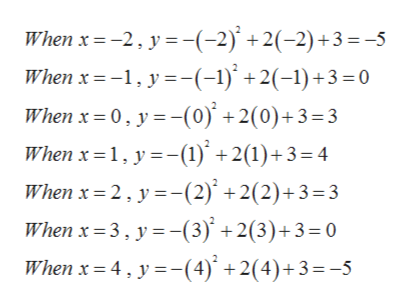# Graph the given equation. First plot the solutions of the equation for the given values of x, and then connect the points with a smooth graph.y = −x2 + 2x + 3x = −2, −1, 0, 1, 2, 3, 4

Question
29 views

Graph the given equation. First plot the solutions of the equation for the given values of x, and then connect the points with a smooth graph.

 y = −x2 + 2x + 3 x = −2, −1, 0, 1, 2, 3, 4
check_circle

Step 1

Given,

Step 2

Now calculating the values of y for ...help_outlineImage TranscriptioncloseWhen x 2 y-(-2) +2(-2)+3 =-5 When x -1 y(-1)+2(-1)+3 0 When x 0, y(0)' +2(0)+3=3 When x 1, y(1) +2(1)+3= 4 When x 2, y(2) +2(2)+3=3 When x 3, y-(3)' +2(3)+3= 0 When x 4, y(4)+2(4)+3=-5 fullscreen

### Want to see the full answer?

See Solution

#### Want to see this answer and more?

Solutions are written by subject experts who are available 24/7. Questions are typically answered within 1 hour.*

See Solution
*Response times may vary by subject and question.
Tagged in

### Other